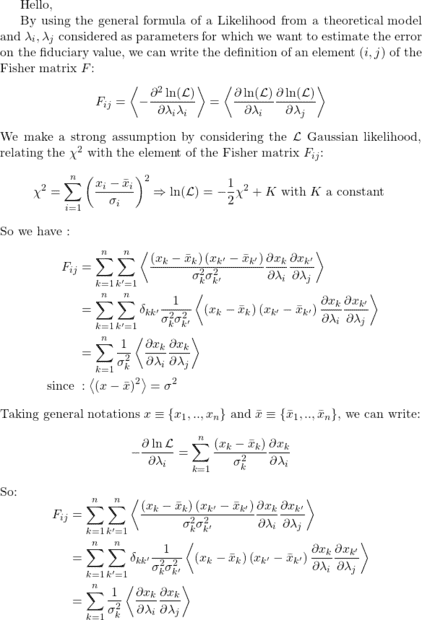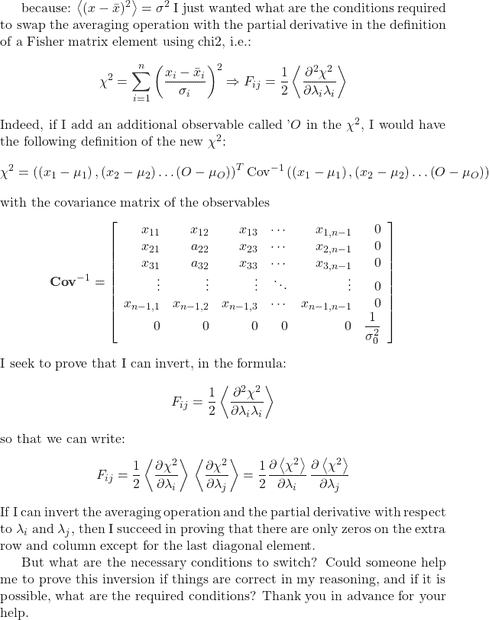# Try to swap between mean and partial derivatives on a product

• A
fab13
TL;DR Summary
I would like to be able to prove that we can swap the mean and partial derivatives on the defintion of a Fisher element matrix : this defintion involves the mean of a product of derivatives on Likelihood. I have also tried to formulate it with the ##chi^2## and the matrix of covariance of observables (noted "Cov" below). All of this is done in the goal that observable big "O" that I introduce is independent and so I have just to sum the extra elements calculated from "O".#### Attachments

$$\langle {\partial \chi^2 \over \partial \lambda_i \lambda_j} \rangle = \langle {\partial \chi^2 \over \partial \lambda_i} \rangle\langle {\partial \chi^2 \over \partial \lambda_j} \rangle$$